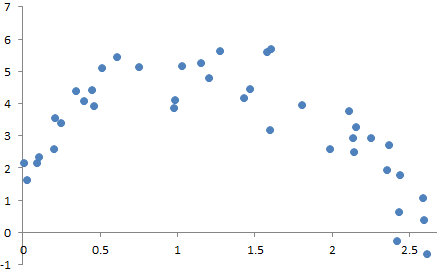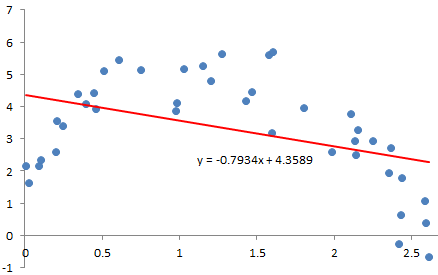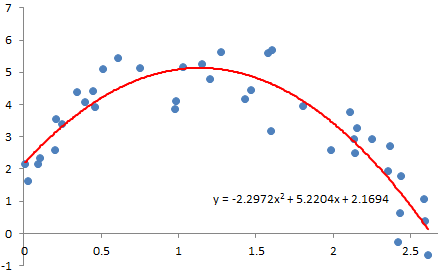## Sunday, September 03, 2023

### Linear and Polynomial Regression in kdb+/q

In this post, I'll describe how you can implement linear and polynomial regression in kdb+/q to determine the equation of a line of best fit (also known as a trendline) through the data on a scatter plot.

Consider the following scatter plot:Our aim is to estimate a function of a line that most closely fits the data.

The vector of estimated polynomial regression coefficients (using ordinary least squares estimation) can be obtained using the following formula (for information about how this formula is derived, see Regression analysis [Wikipedia]):

# ``` b = (XTX)−1XTy ```

This can be translated into q as follows:

```computeRegressionCoefficients:{
xt:flip x;
xt_x:xt mmu x;
xt_x_inv:inv xt_x;
xt_y:xt mmu y;
xt_x_inv mmu xt_y}
```

Linear:
In order to perform linear regression, we have to first create a matrix X with a column of 1s and a column containing the x-values. The output of linear regression will be a vector of 2 coefficients and the equation of the trendline will be of the form: `y = b1x + b0`

```computeLinearRegressionCoefficients:{
computeRegressionCoefficients[flip (1f;x);y]}
```Polynomial:
In order to fit a polynomial line, all we have to do is take the matrix X from the linear regression model and add more columns corresponding to the order of the polynomial desired. For example, for quadratic regression, we will add a column for x2 on the right side of the matrix X. The output of quadratic regression will be a vector of 3 coefficients and the equation of the curve will be of the form: `y = b2x2 + b1x + b0`

```// quadratic
computeRegressionCoefficients[flip (1f;x;x*x);y]}

// cubic
computeCubicRegressionCoefficients:{
computeRegressionCoefficients[flip (1f;x;x*x;x*x*x);y]}

// generalisation of polynomial regression for any order
computePolynomialRegressionCoefficients:{[x;y;order]
computeRegressionCoefficients[flip x xexp/: til order+1;y]}
```Related post:
Matrix Operations in kdb+/q

## Saturday, September 02, 2023

### Python: Printing the Stdout/Stderr of a Subprocess

This is how you can run a subprocess in python and print out its stdout and stderr:

```import subprocess

proc = subprocess.Popen(["/path/to/myscript", "arg1", "arg2"],
stderr=subprocess.STDOUT, stdout=subprocess.PIPE)
for line in proc.stdout:
print(line.decode().rstrip())
proc.wait()
if proc.returncode != 0:
print("Command failed with status:", proc.returncode)
```

Check out the `subprocess` documentation for more information.

Related post:
Python Cheat Sheet